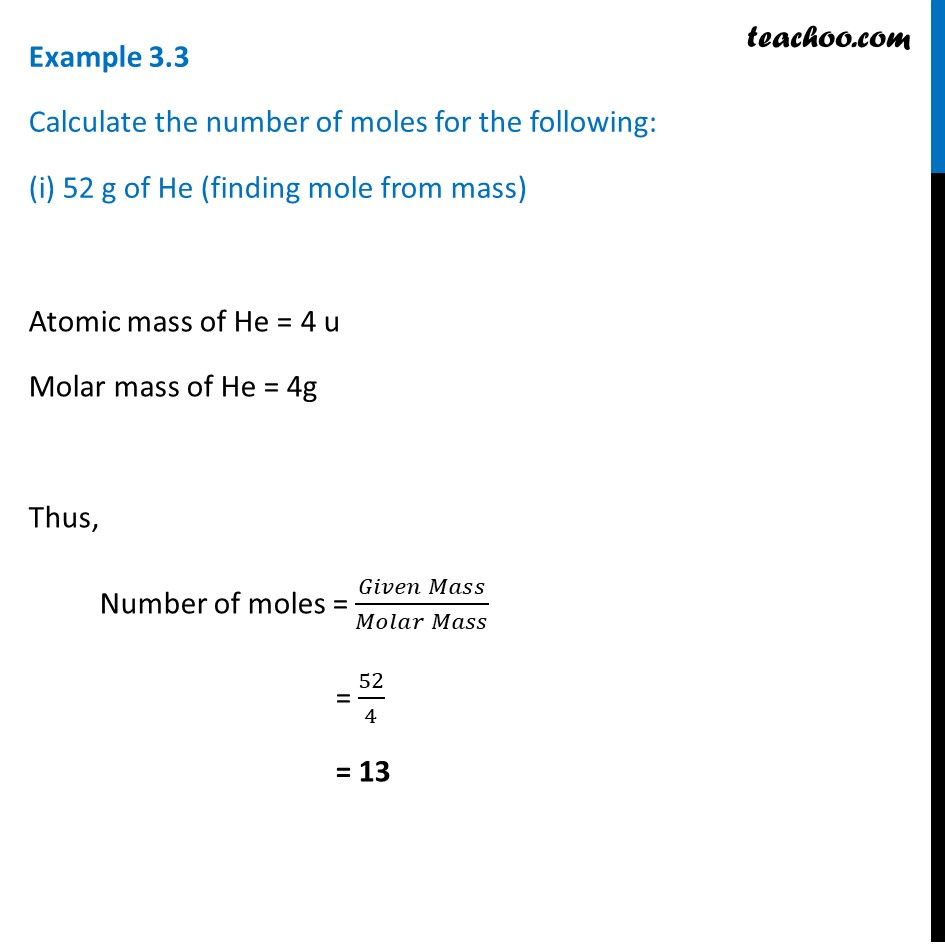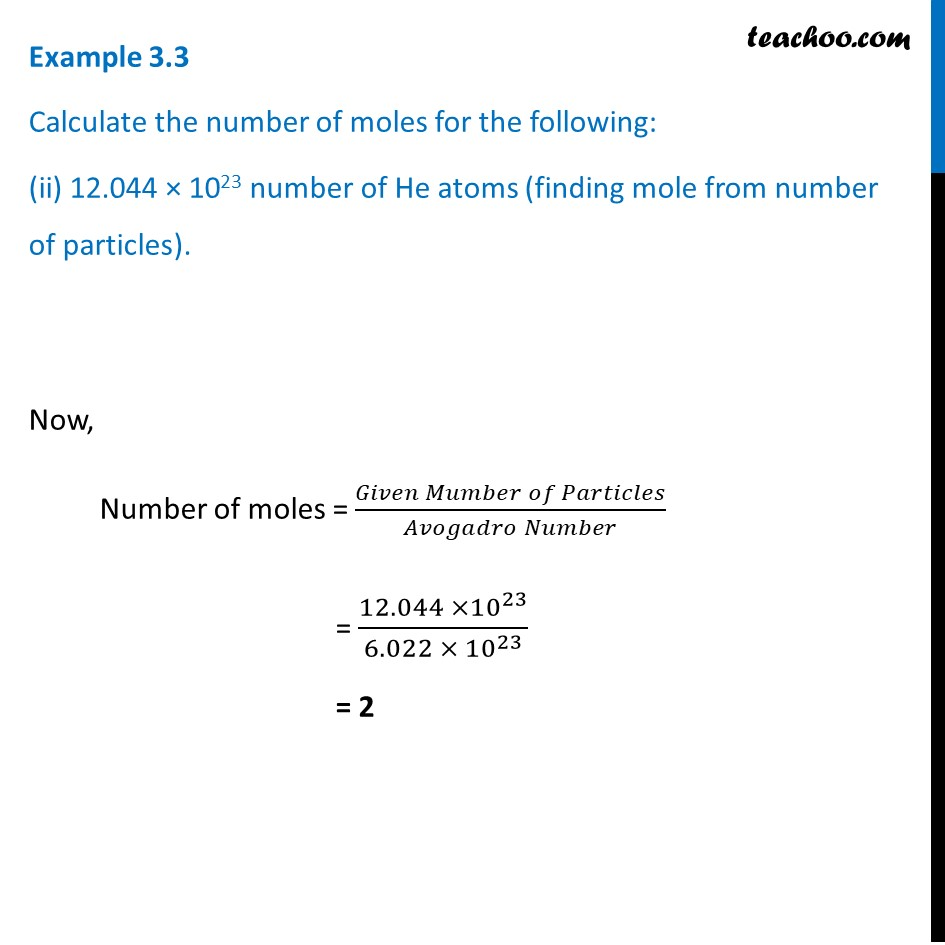1. Class 9
2. Chapter 3 Class 9 - Atoms And Molecules (Term 2)
3. Examples from NCERT Book

Transcript

Example 3.3 Calculate the number of moles for the following: (i) 52 g of He (finding mole from mass) Atomic mass of He = 4 u Molar mass of He = 4g Thus, Number of moles = (𝐺𝑖𝑣𝑒𝑛 𝑀𝑎𝑠𝑠)/(𝑀𝑜𝑙𝑎𝑟 𝑀𝑎𝑠𝑠) = 52/4 = 13 Example 3.3 Calculate the number of moles for the following: (ii) 12.044 × 1023 number of He atoms (finding mole from number of particles). Now, Number of moles = (𝐺𝑖𝑣𝑒𝑛 𝑀𝑢𝑚𝑏𝑒𝑟 𝑜𝑓 𝑃𝑎𝑟𝑡𝑖𝑐𝑙𝑒𝑠)/(𝐴𝑣𝑜𝑔𝑎𝑑𝑟𝑜 𝑁𝑢𝑚𝑏𝑒𝑟) = (12.044 ×10^23)/(6.022 × 10^23 ) = 2

Examples from NCERT Book

Class 9
Chapter 3 Class 9 - Atoms And Molecules (Term 2)